MATLAB Function Referencedel2

Discrete Laplacian

Syntax

• ```L = del2(U)
L = del2(U,h)
L = del2(U,hx,hy)
L = del2(U,hx,hy,hz,...)
```

Definition

If the matrix `U` is regarded as a function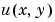evaluated at the point on a square grid, then `4*del2(U)` is a finite difference approximation of Laplace's differential operator applied to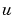, that is:

•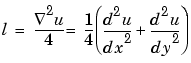where:

•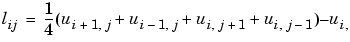in the interior. On the edges, the same formula is applied to a cubic extrapolation.

For functions of more variables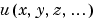, `del2(U)` is an approximation,

•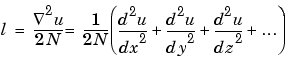where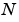is the number of variables in.

Description

```L = del2(U) ``` where `U` is a rectangular array is a discrete approximation ofThe matrix `L` is the same size as `U` with each element equal to the difference between an element of `U` and the average of its four neighbors.

```-L = del2(U) ``` when `U` is an multidimensional array, returns an approximation of

•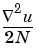whereis `ndims(u)`.

```L = del2(U,h) ``` where `H` is a scalar uses `H` as the spacing between points in each direction (`h=1` by default).

```L = del2(U,hx,hy) ``` when `U` is a rectangular array, uses the spacing specified by `hx` and `hy`. If `hx` is a scalar, it gives the spacing between points in the x-direction. If `hx` is a vector, it must be of length `size(u,2)` and specifies the x-coordinates of the points. Similarly, if `hy` is a scalar, it gives the spacing between points in the y-direction. If `hy` is a vector, it must be of length `size(u,1)` and specifies the y-coordinates of the points.

```L = del2(U,hx,hy,hz,...) ``` where `U` is multidimensional uses the spacing given by `hx`, `hy`, `hz`, ...

Examples

The function

•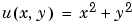has

•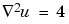For this function, `4*del2(U)` is also `4`.

• ```[x,y] = meshgrid(-4:4,-3:3);
U = x.*x+y.*y
U =
25     18     13     10      9     10     13     18     25
20     13      8      5      4      5      8     13     20
17     10      5      2      1      2      5     10     17
16      9      4      1      0      1      4      9     16
17     10      5      2      1      2      5     10     17
20     13      8      5      4      5      8     13     20
25     18     13     10      9     10     13     18     25
```

• ```V = 4*del2(U)
V =
4      4      4      4      4      4      4      4      4
4      4      4      4      4      4      4      4      4
4      4      4      4      4      4      4      4      4
4      4      4      4      4      4      4      4      4
4      4      4      4      4      4      4      4      4
4      4      4      4      4      4      4      4      4
4      4      4      4      4      4      4      4      4
```

See Also

`diff`, `gradient`

© 1994-2005 The MathWorks, Inc.